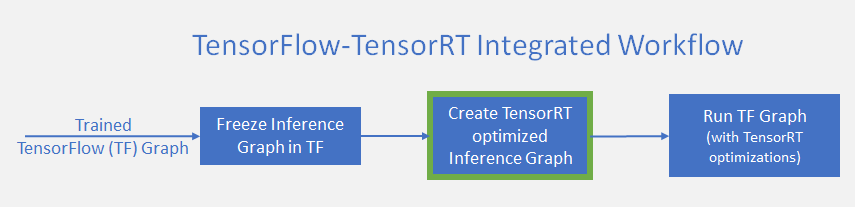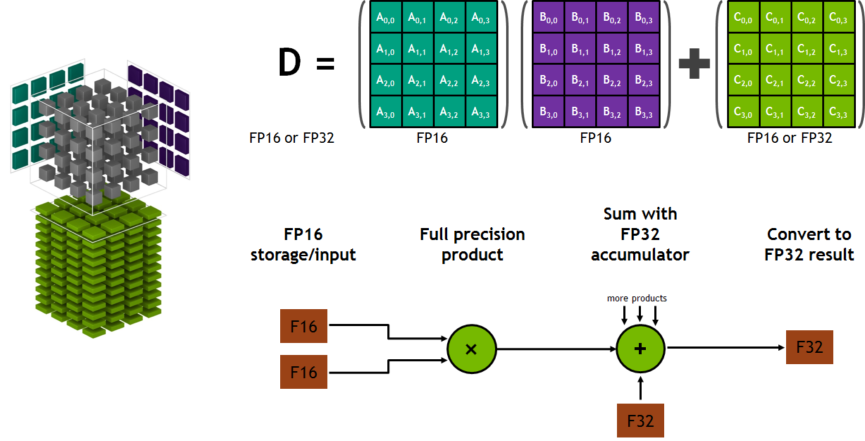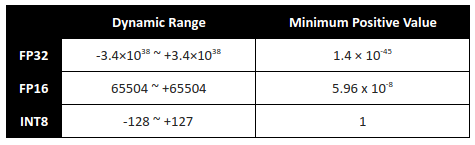Community ·Speed up TensorFlow Inference on GPUs with TensorRT
April 18, 2018
Posted by Siddharth Sharma — Technical Product Marketing Manager, NVidia;
Sami Kama — Deep Learning Developer Technologist, NVidia;
Julie Bernauer — Pursuit Engineering Solution Architect, NVidia;

### Overview

TensorFlow remains the most popular deep learning framework today, with tens of thousands of users worldwide. NVIDIA® TensorRT™ is a deep learning platform that optimizes neural network models and speeds up for inference across GPU-accelerated platforms running in the datacenter, embedded and automotive devices. We are excited about the integration of TensorFlow with TensorRT, which seems a natural fit, particularly as NVIDIA provides platforms well-suited to accelerate TensorFlow. This enables TensorFlow users with extremely high inference performance plus a near transparent workflow when using TensorRT.Figure 1. TensorRT optimizes trained neural network models to produce deployment-ready runtime inference engines.
TensorRT performs several important transformations and optimizations to the neural network graph (Fig 2). First, layers with unused output are eliminated to avoid unnecessary computation. Next, where possible convolution, bias, and ReLU layers are fused to form a single layer. Another transformation is horizontal layer fusion, or layer aggregation, along with the required division of aggregated layers to their respective output. Horizontal layer fusion improves performance by combining layers that take the same source tensor and apply the same operations with similar parameters. Note that these graph optimizations do not change the underlying computation in the graph: instead, they look to restructure the graph to perform the operations much faster and more efficiently.Figure 2 (a): An example convolutional neural network with multiple convolutional and activation layers. (b) TensorRT’s vertical and horizontal layer fusion and layer elimination optimizations simplify the GoogLeNet Inception module graph, reducing computation and memory overhead.
If you were already using TensorRT with TensorFlow models, you knew that applying TensorRT optimizations used to require exporting the trained TensorFlow graph. You also needed to manually import certain unsupported TensorFlow layers, and then run the complete graph in TensorRT. You should not need to do that for most cases any more. In the new workflow, you use a simple API to apply powerful FP16 and INT8 optimizations using TensorRT from within TensorFlow. Existing TensorFlow programs require only a couple of new lines of code to apply these optimizations.

TensorRT sped up TensorFlow inference by 8x for low latency runs of the ResNet-50 benchmark. These performance improvements cost only a few lines of additional code and work with the TensorFlow 1.7 release and later. In this article we will describe the new workflow and APIs to help you get started with it.

### Applying TensorRT optimizations to TensorFlow graphs

Adding TensorRT to the TensorFlow inference workflow involves an additional step, shown in Figure 3. In this step (highlighted in green), TensorRT builds an optimized inference graph from a frozen TensorFlow graph.Figure 3: Workflow Diagram when using TensorRT within TensorFlow
To accomplish this, TensorRT takes the frozen TensorFlow graph and parses it to select sub-graphs that it can optimize. It then applies optimizations to the subgraphs and replaces them with TensorRT nodes in the original TensorFlow graph leaving the remaining graph unchanged. During inference, TensorFlow executes the complete graph calling TensorRT to run the TensorRT optimized nodes. With this approach, developers can continue to use the flexible TensorFlow feature set with the optimizations of TensorRT.

Let’s look at an example of a graph with three segments, A, B, and C. TensorRT optimizes Segment B, then replaces it with a single node. During inference, TensorFlow executes A, calls TensorRT to execute B, and then TensorFlow executes C. From a user’s perspective, you continue to work in TensorFlow as earlier.

TensorRT optimizes the largest sub-graphs possible in the TensorFlow graph. The more compute in the subgraph, the greater benefit obtained from TensorRT. You want most of the graph optimized and replaced with the fewest number of TensorRT nodes for best performance. Based on the operations in your graph, it’s possible that the final graph might have more than one TensorRT nodes. With the TensorFlow API, you can specify the minimum number of the nodes in a sub-graph for it to be converted to a TensorRT node. Any sub-graph with less than the specified set number of nodes will not be converted to TensorRT engines even if it is compatible with TensorRT. This can be useful for models containing small compatible sub-graphs separated by incompatible nodes, in turn leading to tiny TensorRT engines.

Let’s look at how to implement the workflow in more detail.

### Using New TensorFlow APIs

The new TensorFlow API enables straightforward implementation of TensorRT optimizations with a couple of lines of new code. First, specify the fraction of available GPU memory that TensorFlow is allowed to use, the remaining memory being available for TensorRT engines. This can be done with the new `per_process_gpu_memory_fraction` parameter of the `GPUOptions` function. This parameter needs to be set the first time the TensorFlow-TensorRT process starts. For example, setting `per_process_gpu_memory_fraction` to 0.67 allocates 67% of GPU memory for TensorFlow and the remaining third for TensorRT engines.
``````gpu_options = tf.GPUOptions(per_process_gpu_memory_fraction =
0 < memory_for_TensorFlow < 1)``````
The next step is letting TensorRT analyze the TensorFlow graph, apply optimizations, and replace subgraphs with TensorRT nodes. You apply TensorRT optimizations to the frozen graph with the new `create_inference_graph function`. This function uses a frozen TensorFlow graph as input, then returns an optimized graph with TensorRT nodes, as shown in the following code snippet:
``````trt_graph = trt.create_inference_graph(
input_graph_def = frozen_graph_def,
outputs = output_node_name,
max_batch_size=batch_size,
max_workspace_size_bytes=workspace_size,
precision_mode=precision,
minimum_segment_size=3)``````
Let’s look at the function’s parameters:

`input_graph_def`: frozen TensorFlow graph

`outputs`: list of strings with names of output nodes e.g.[“`resnet_v1_50/predictions/Reshape_1`”]

`max_batch_size`: integer, size of input batch e.g. 16

`max_workspace_size_bytes`: integer, maximum GPU memory size available for TensorRT

`precision_mode`: string, allowed values “FP32”, “FP16” or “INT8”

`minimum_segment_size`: integer (default = 3), control min number of nodes in a sub-graph for TensorRT engine to be created

The `per_process_gpu_memory_fraction` and `max_workspace_size_bytes` parameters should be used together to split GPU memory available between TensorFlow and TensorRT to get providing best overall application performance.To maximize inference performance, you might want to give TensorRT slightly more memory than what it needs, giving TensorFlow the rest. For example, if you set the `per_process_gpu_memory_fraction` parameter to ( 12–4 ) / 12 = 0.67, then setting max_workspace_size_bytes parameter to 4000000000 for a 12GB GPU allocates ~4GB for the TensorRT engines. Again, finding the most optimum memory split is application dependent and might require some iteration.

### Using TensorBoard to Visualize Optimized Graphs

TensorBoard enables us to visualize the changes to the ResNet-50 node graph once TensorRT optimizations are applied in TensorBoard. Figure 4 shows that TensorRT optimizes almost the complete graph, replacing it with a single node titled “my_trt_op0” (highlighted in red). Depending on the layers and operations in your model, TensorRT nodes replace portions of your model due to optimizations. The box titled “conv1” isn’t actually a convolution layer; it’s really a transpose operation from NHWC to NCHW.Figure 4. (a) ResNet-50 graph in TensorBoard (b) ResNet-50 after TensorRT optimizations have been applied and the sub-graph replaced with a TensorRT node.

### Using Tensor Cores on Volta GPUs

Using half-precision (also called FP16) arithmetic reduces memory usage of the neural network compared with FP32 or FP64. FP16 enables deployment of larger networks while taking less time than FP32 or FP64. NVIDIA’s Volta architecture incorporates hardware matrix math accelerators known as Tensor Cores. Tensor Cores provide a 4x4x4 matrix processing array which performs the operation D = A * B + C, where A, B, C and D are 4×4 matrices. Figure 5 shows how this works. The matrix multiply inputs A and B are FP16 matrices, while the accumulation matrices C and D may be FP16 or FP32 matrices.Fig. 5: Matrix processing operations on Tensor Cores
TensorRT automatically uses hardware Tensor Cores when detected for inference when using FP16 math. Tensor Cores offer peak performance about an order of magnitude faster on the NVIDIA Tesla V100 than double-precision (FP64) while throughput improves up to 4 times faster than single-precision (FP32). Just use “FP16” as value for the `precision_mode` parameter in the `create_inference_graph` function to enable half precision, as shown below. `getNetwork()` is a helper function that reads the frozen network from the protobuf file and returns a` tf.GraphDef() `of the network.
``````trt_graph = trt.create_inference_graph(
getNetwork(network_file_name),
outputs,
max_batch_size=batch_size,
max_workspace_size_bytes=workspace_size,
precision_mode=”FP16")``````
Figure 6 shows ResNet-50 performing 8 times faster under 7 ms latency with the TensorFlow-TensorRT integration using NVIDIA Volta Tensor Cores versus running TensorFlow only on the same hardware.Fig. 6: ResNet-50 inference throughput performance

### Inference using INT8 precision

Performing inference using INT8 precision further improves computation speed and places lower requirements on bandwidth. The reduced dynamic range makes it challenging to represent weights and activations of neural networks.TensorRT provides capabilities to take models trained in single (FP32) and half (FP16) precision and convert them for deployment with INT8 quantizations while minimizing accuracy loss. Converting models for deployment with INT8 requires calibrating the trained FP32 model before applying the TensorRT optimizations described earlier. The workflow changes to incorporate a calibration step prior to creating the TensorRT optimized inference graph, as shown in Figure 7:Figure 7. Workflow incorporating INT8 inference
First use the `create_inference_graph` function, setting the `precision_mode` parameter set to “INT8” to calibrate the model. The output of this function is a frozen TensorFlow graph ready for calibration.
``````trt_graph = trt.create_inference_graph(
getNetwork(network_file_name),
outputs,
max_batch_size=batch_size,
max_workspace_size_bytes=workspace_size,
precision_mode=”INT8")``````
Now run the calibration graph with calibration data. TensorRT uses the distribution of node data to quantize weights for the nodes. It’s imperative you use calibration data closely reflecting the distribution of the problem dataset in production. We suggest checking for error accumulation during inference when first using models calibrated with INT8. The `minimum_segment_size `parameter can help tune the optimized graph to minimize quantization-errors. Using `minimum_segment_size,` you can change the minimum number of nodes in the optimized INT8 engines to change the final optimized graph to fine tune result accuracy.

After executing the graph on calibration data, apply TensorRT optimizations to the calibration graph with the `calib_graph_to_infer_graph` function. This function also replaces the TensorFlow subgraph with a TensorRT node optimized for INT8. The output of the function is a frozen TensorFlow graph that can be used for inference as usual.
``trt_graph=trt.calib_graph_to_infer_graph(calibGraph)``
All it takes are these two commands to enable INT8 precision inference with your TensorFlow model.

If you want to check out the examples shown here, check out code required to run these examples at https://developer.download.nvidia.com/devblogs/tftrt_sample.tar.xz

### Availability

We expect that integrating TensorRT with TensorFlow will yield the highest performance possible when using NVIDIA GPUs while maintaining the ease and flexibility of TensorFlow. NVIDIA continues to work closely with the Google TensorFlow team to further enhance these integration capabilities. Developers will automatically benefit from updates as TensorRT supports more networks, without needing to change existing code.

Find instructions on how to get started today at: https://www.tensorflow.org/install/install_linux

In the near future, we expect the standard pip install process to work as well. Stay tuned!

We believe you’ll see substantial benefits to integrating TensorRT with TensorFlow when using GPUs. You can find more information on TensorFlow at https://www.tensorflow.org/.

Additional information on TensorRT can be found on NVIDIA’s TensorRT page at https://developer.nvidia.com/tensorrt.
Next postPosted by Siddharth Sharma — Technical Product Marketing Manager, NVidia;
Sami Kama — Deep Learning Developer Technologist, NVidia;
Julie Bernauer — Pursuit Engineering Solution Architect, NVidia;